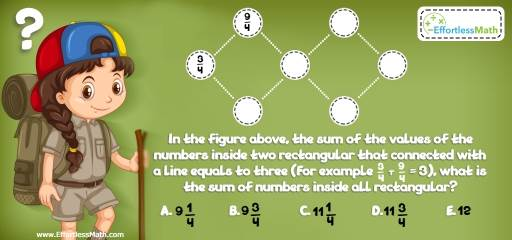# Number Properties Puzzle – Challenge 3

A perfect math puzzle for those who love to be challenged by mathematics and critical thinking math problems.## Challenge:

In the figure above, the sum of the values of the numbers inside two rectangular that is connected with a line equals three (For example $$\frac{3}{4 }+\frac{ 9}{4} = 3$$), what is the sum of numbers inside all rectangular?

A- $$9\frac{1}{4}$$

B- $$9\frac{3}{4}$$

C- $$11\frac{1}{4}$$

D- $$11\frac{3}{4}$$

E- $$12$$

Best Algebra Prep Resource

### The Absolute Best Bookto ChallengeYour Smart Student!

Write the values of all rectangular and add them.
$$\frac{3}{4} + \frac{9}{4} + \frac{3}{4} + \frac{9}{4} +\frac{ 3}{4 }+ \frac{9}{4 }+ \frac{3}{4 }= 9 \frac{3}{4}$$The Absolute Best Books to Ace Algebra

### What people say about "Number Properties Puzzle – Challenge 3 - Effortless Math: We Help Students Learn to LOVE Mathematics"?

No one replied yet.

X
45% OFF

Limited time only!

Save Over 45%

SAVE $40 It was$89.99 now it is \$49.99